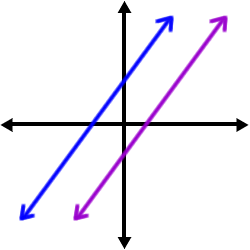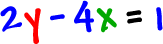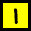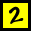This is easy!  Look at these parallel lines:What do they have in common?

 The same slope!

So, two lines are parallel if they have the same slope.

The problems in this section (and the next) are cool because they pull together a bunch of stuff I've shown you.

Check it out:

Let's find the equation of the line that passes through the point

 ( -3 , 5 ) and is parallel to the line.

* To get the equation of a line we need:a point -- which we havea slope -- which we can get from(since they are parallel, they'll have the same slope.)